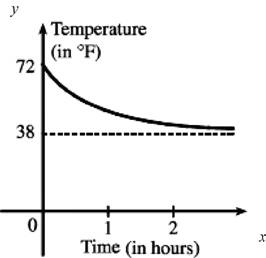# The initial rate of change of temperature greater or less than the rate of change after an hour.### Single Variable Calculus: Concepts...

4th Edition
James Stewart
Publisher: Cengage Learning
ISBN: 9781337687805### Single Variable Calculus: Concepts...

4th Edition
James Stewart
Publisher: Cengage Learning
ISBN: 9781337687805

#### Solutions

Chapter 2.6, Problem 41E
To determine

## To find: The initial rate of change of temperature greater or less than the rate of change after an hour.

Expert Solution

The initial rate of change is greater than the rate of change after an hour.

### Explanation of Solution

Graph:

Sketch the rough graph as shown in Figure 1.From the graph, the room temperature is 72° and the refrigerator temperature is 38°.

Thus, the initial rate of change is greater than the rate of change after an hour.

### Have a homework question?

Subscribe to bartleby learn! Ask subject matter experts 30 homework questions each month. Plus, you’ll have access to millions of step-by-step textbook answers!